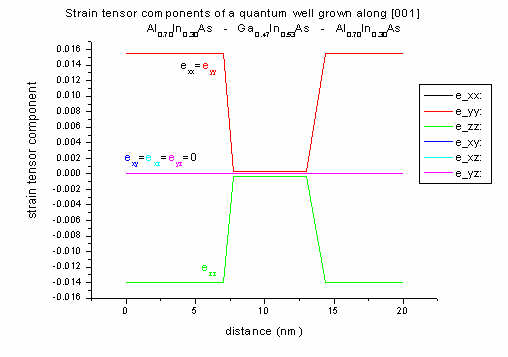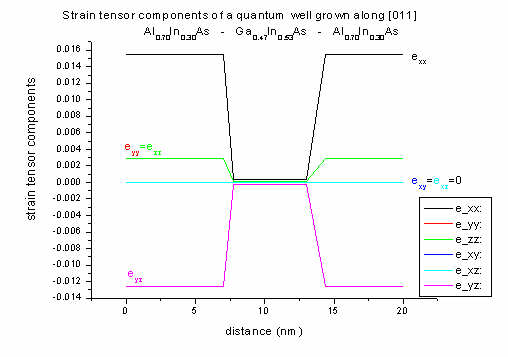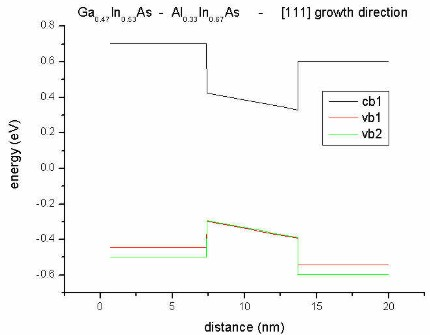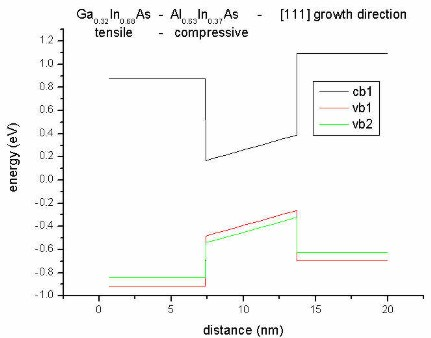nextnano.com  Tool: nextnano++  Tool: nextnano³  Download | Search | Copyright | Publications  * password protected nextnano³ software1D piezo

# nextnano3 - Tutorial

## Piezoelectric fields due to strain (Quantum well)

Information on strain can also be found in the FAQ section and in the strain tutorial.

Author: Stefan Birner

If you want to obtain the input files that are used within this tutorial, please check if you can find them in the installation directory.
If you cannot find them, please submit a Support Ticket.
```-> 1Dpiezo_Al0.70In0.30As_In0.53Ga0.47As_100_nn3_growth_along_z_direction.in - ```input file for the nextnano3 software
```   1Dpiezo_Al0.70In0.30As_In0.53Ga0.47As_311_nn3_growth_along_z_direction.in - ```input file for the nextnano3 software
`   1Dpiezo_Al0.70In0.30As_In0.53Ga0.47As_100_nn3.in / *_nnp.in               - `input file for the nextnano3 and nextnano++ software```    1Dpiezo_Al0.70In0.30As_In0.53Ga0.47As_311_nn3.in / *_nnp.in               - ```input file for the nextnano3 and nextnano++ software```    1Dpiezo_Al0.33In0.67As_In0.32Ga0.68As_111_nn3.in / *_nnp.in               - ```input file for the nextnano3 and nextnano++ software

Input files for nextnano.MSB and nextnano.NEGF software for this tutorial are also available.

## Piezoelectric fields

Piezoelectricity is due to the ionicity of III-V semiconductor compounds.
Si and Ge have purely covalent bonds whereas the bonds of III-V compounds are partially ionic (heteropolar) because the electrons spend on average more time next to the (negatively charged) anions.

Zinc-blende structures are piezoelectric materials. Off-diagonal strain tensor components (jk) induce a polarization given by
Pis = 2 e14 epsilonjk        (epsilon = strain tensor)
where Ps is the induced polarization, e14 is the piezoelectric constant and epsilonjk is a symmetrized strain component.
However, diagonal strains (epsilonxx, epsilonyy, epsilonzz) do not induce a polarization (i.e. e11=0).
A strained layer superlattice with a  growth direction will induce only diagonal strains; but with any other growth direction, off-diagonal strains also occur.
Thus -growth axis strained-layer heterostructures will not have strain-induced polarization fields, but strained-layer heterostructures with any other growth direction will have these polarization fields.
Because one of the constituent materials is in biaxial tension and the other is in biaxial compression, the polarization vector changes sign at the interface.
For a  growth axis, Ps is parallel to the growth axis, for a  growth axis, Ps is in the interface plane; for a  growth axis, Ps vanishes.
For a general growth axis, Ps has components both parallel and perpendicular to the growth axis.
The resulting interface charge density is given by   rho = - div Ps.
`==> `Text taken from D.L. Smith, C. Mailhiot, Rev. Mod. Phys. 62 (1), 173 (1990)

Thus we want to test this on a quantum well structure consisting of Ga0.47In0.53As (quantum well) and AlxIn1-xAs (barrier).

We will try 3 growth directions for Al0.70In0.30As.

•  - no strain-induced polarization fields (E=0)
•  - polarization vector Ps lies in the interface plane
•  - polarization vector Ps is perpendicular to interface and parallel to growth direction

(A superlattice grown in  direction has a different point group symmetry, namely C3v, than  growth which is D2d.)

We will also try growth direction  with different alloy compositions for the barrier resulting in different piezoelectric constants for each barrier material.

• Al0.63In0.37As
• Al0.33In0.67As

Ga0.47In0.53As/Al0.48In0.52As is lattice matched to InP.
By proper choice of alloy composition of AlxIn1-xAs, one can subject this layer to either tensile or compressive strain when grown pseudomorphic on InP leading to a splitting in light hole (lh) and heavy hole (hh) bands and also changing their order.

Okay, let's compare the growth directions for a Ga0.47In0.53As - Al0.70In0.30As quantum well.

• along  direction
```==> 1Dpiezo_Al0.70In0.30As_In0.53Ga0.47As_100_nn3_growth_along_z_direction.in```
Splitting due to strain: The heavy hole band (vb1) is lower than the light hole band (vb2) of Al0.70In0.30As (barrier) due to tensile strain.
Due to the  growth direction, no piezoelectric charges are present.Conduction
and
valence bands
alongStrain
along 

 growth direction: The well region is lattice matched to InP, therefore strain is zero. Only the barrier region is subject to strain. The off-diagonal strain tensor components are all zero (exy=exz=eyz=0) as we have  growth direction. Al0.70In0.30As has a smaller lattice constant than the substrate material InP (lattice mismatch 1.55 %) leading to tensile strain.

In 1D strain can be calculated analytically along  growth direction:
(a = lattice constant, c11, c12 = elastic constants):
Biaxial strain (in plane of interface):
exx = eyy = ( asubstrate - alayer ) / alayer = 0.0155   (1.55 % lattice mismatch)
Uniaxial strain (perpendicular to interface):
ezz = - 2 (c12/c11) exx = - 0.014

For  growth direction, crystal and simulation system coincide. Thus the strain given in the crystal coordinate system is equal to the strain given in the simulation system.

• along  direction
changes in input file:
`hkl-z-direction-zb = 0  1  1  ! `Miller indices of z coordinate axis [0 0 1]``` hkl-y-direction-zb = 0  1 -1  ! ```Miller indices of y coordinate axis [0 1 0]
There's no effect due to piezoelectric charges as the polarization vector is directed along the interface (perpendicular to growth direction).Conduction
and
valence bands
alongStrain
along 

 growth direction: The well region is lattice matched to InP, therefore strain is zero. Only the barrier region is subject to strain.
exx again can be calculated analytically as above with the same result. The off-diagonal strain components that include x are zero (exy=exz=0). Due to shear strain in the (y,z) plane, eyz is not zero.
Al0.70In0.30As has a smaller lattice constant than the substrate material InP (lattice mismatch 1.55 %) leading to tensile strain.

In 1D layered heterostructures, strain can be calculated analytically along  growth direction (see FAQ section). The strain tensor components can be printed out with respect to the crystal coordinate system and simulation coordinate system (see keyword``` \$output-strain```).

For  growth direction, crystal and simulation system do not coincide anymore. Thus the strain given in the crystal coordinate system is not equal to the strain given in the simulation system anymore.
In the simulation system all off-diagonal components of the strain tensor are zero (exy,sim=exz,sim=eyz,sim=0) and eyy,sim=ezz,sim.

• along  direction
changes in input file:
`hkl-z-direction-zb = 1 1 1  ! `Miller indices of z coordinate axis [1  1  1]``` hkl-y-direction-zb = 0 1 -1 ! ```Miller indices of y coordinate axis [0  1 -1]
The polarization vector due to piezoelectric charges is directed along the  growth direction leading to a slope in the conduction and valence bands.Conduction
and
valence bands
alongStrain
along 

 growth direction: The well region is lattice matched to InP, therefore strain is zero. Only the barrier region is subject to strain.
In 1D strain can be calculated analytically along  growth direction (see FAQ section).
The off-diagonal strain components (shear strain) are all equal (exy=exz=eyz).

Al0.70In0.30As has a smaller lattice constant than the substrate material InP (lattice mismatch 1.55 %) leading to tensile strain.

For  growth direction, crystal and simulation system do not coincide either. Again, the strain given in the crystal coordinate system is not equal to the strain given in the simulation system.
In the simulation system all off-diagonal components of the strain tensor are again zero (exy,sim=exz,sim=eyz,sim=0) and eyy,sim=ezz,sim.
Note: For  growth direction eyz,sim=0 but all other off-diagonal compontents are not zero anymore. In the simulation system, for any growth direction, eyy,sim=ezz,sim=exx,sim=exx,sim[hkl].

For the pictures above, the strain tensor components are plotted with respect to the crystal coordinate system, not simulation coordinate system.

Discussion of piezoelectric field
For the  case the polarization vector is along . As expected we get an electric field. For  the polarization vector lies in the interface (no resulting E field) and for  there isn't any polarization verctor.

( growth: If you change the piezoelectric constant e14 in the` database_nn3.in `file to zero, you won't get a slope and the picture looks as for the  case. If you change the signs of these constants from negative to positive, the slope would be reversed. The direction depends on the sign, e.g. positive from In (cation) to As (anion) along .)
You can also switch of piezoelectricity using the flag``` piezo-constants-zero = yes ```in``` \$numeric-control```.

• along  direction
Again we take the  direction but this time we take lattice matched Ga0.47In0.53As and Al0.48In0.52As (lattice matched to InP) herby avoiding any strain. Thus we do not get any heavy hole/light hole splitting. Without strain we even don't get piezoelectric charges and thus no slope.• along  direction
Here, we change the alloy composition of the barrier.
(strain calculation: pseudomorphic on InP substrate: ``` homogeneous-strain```)
- biaxial compression for            Al0.33In0.67As
- biaxial tension for   Al0.63In0.37As
- still no strain for the well

Now the electric field resulting from piezoelectric charges changes its direction in the two cases.
The heavy hole (vb1) / light hole (vb2) splitting is also different in these two cases!biaxial compression
for
Al0.33In0.67Asbiaxial tension for:
Al0.63In0.37As

• along  direction
Our substrate is InP.
We now combine compressive (Ga0.32In0.68As) and tensile (Al0.63In0.37As) strain. Now for both materials, heavy hole (vb1) and light hole (vb2) bands split but into opposite directions.biaxial tension for:
Al0.63In0.37As
(barrier)

biaxial compression
for:
Ga0.32In0.68As
(well)

• along  direction
`==> 1Dpiezo_Al0.33In0.67As_In0.32Ga0.68As_111_nn3.in`
Our substrate is InP.
We now combine tensile (Ga0.68In0.32As) and compressive (Al0.33In0.67As) strain. Now for both materials, heavy hole (vb1) and light hole (vb2) bands split again but into opposite directions and into opposite directions compared to the previous pictures.biaxial compression for:
Al0.33In0.67As
(barrier)

biaxial tension
for:
Ga0.68In0.32As
(well)

Step 1: Quantum well structure - Piezoelectric fields

1. The structure is grown pseudomorphic on an InP substrate.
2. We perform a one-dimensional simulation.
3. Just a reminder: If you need additional information about the keywords and their specifiers, you can look it up here.
4. The heterostructure looks like this:

```!    barrier            well             barrier!       1                 2                 3    !! Al(x)In(1-x)As    In(x)Ga(1-x)As    Al(x)In(1-x)As!       7                 6                 7           nm!___________________________________________________```
5. The structure has no doping.
6. Output
- The band structure will be saved into the directory``` band_profile/ ```- The densities will be saved into` densities/`- The strain will be saved into``` strain/ ```- The material parameters will be saved into` material_parameters/`
7. You should be able to modify the appropriate lines in the input file ``` 1Dpiezo.in ```in order to reproduce the results shown above.
```  \$domain-coordinates  ... \$end_domain-coordinates  \$alloy-function  ... \$end_alloy-function```
 If you need support, or input files that are not included in the installation folder, or if you have suggestions on how we can improve our website or tutorials, please submit a Support Ticket.